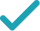Type a math problemSolve for x (complex solution)Steps Using the Quadratic Formula
Steps for Completing the Square
Steps Using Direct Factoring Method
All equations of the form can be solved using the quadratic formula: . The quadratic formula gives two solutions, one when is addition and one when it is subtraction.
This equation is in standard form: . Substitute for , for , and for in the quadratic formula, .
Square .
Multiply times .
Multiply times .
Take the square root of .
Multiply times .
Now solve the equation when is plus. Add to .
Divide by .
Now solve the equation when is minus. Subtract from .
Divide by .
The equation is now solved.
Graph
2x^{2}+12x+40=0
All equations of the form ax^{2}+bx+c=0 can be solved using the quadratic formula: \frac{-b±\sqrt{b^{2}-4ac}}{2a}. The quadratic formula gives two solutions, one when ± is addition and one when it is subtraction.
x=\frac{-12±\sqrt{12^{2}-4\times 2\times 40}}{2\times 2}
This equation is in standard form: ax^{2}+bx+c=0. Substitute 2 for a, 12 for b, and 40 for c in the quadratic formula, \frac{-b±\sqrt{b^{2}-4ac}}{2a}.
x=\frac{-12±\sqrt{144-4\times 2\times 40}}{2\times 2}
Square 12.
x=\frac{-12±\sqrt{144-8\times 40}}{2\times 2}
Multiply -4 times 2.
x=\frac{-12±\sqrt{144-320}}{2\times 2}
Multiply -8 times 40.
x=\frac{-12±\sqrt{-176}}{2\times 2}
x=\frac{-12±4\sqrt{11}i}{2\times 2}
Take the square root of -176.
x=\frac{-12±4\sqrt{11}i}{4}
Multiply 2 times 2.
x=\frac{-12+4\sqrt{11}i}{4}
Now solve the equation x=\frac{-12±4\sqrt{11}i}{4} when ± is plus. Add -12 to 4i\sqrt{11}\approx 13.266499161i.
x=-3+\sqrt{11}i
Divide -12+4i\sqrt{11}\approx -12+13.266499161i by 4.
x=\frac{-4\sqrt{11}i-12}{4}
Now solve the equation x=\frac{-12±4\sqrt{11}i}{4} when ± is minus. Subtract 4i\sqrt{11}\approx 13.266499161i from -12.
x=-\sqrt{11}i-3
Divide -12-4i\sqrt{11}\approx -12-13.266499161i by 4.
x=-3+\sqrt{11}i x=-\sqrt{11}i-3
The equation is now solved.
2x^{2}+12x+40=0
Quadratic equations such as this one can be solved by completing the square. In order to complete the square, the equation must first be in the form x^{2}+bx=c.
2x^{2}+12x+40-40=-40
Subtract 40 from both sides of the equation.
2x^{2}+12x=-40
Subtracting 40 from itself leaves 0.
\frac{2x^{2}+12x}{2}=\frac{-40}{2}
Divide both sides by 2.
x^{2}+\frac{12}{2}x=\frac{-40}{2}
Dividing by 2 undoes the multiplication by 2.
x^{2}+6x=\frac{-40}{2}
Divide 12 by 2.
x^{2}+6x=-20
Divide -40 by 2.
x^{2}+6x+3^{2}=-20+3^{2}
Divide 6, the coefficient of the x term, by 2 to get 3. Then add the square of 3 to both sides of the equation. This step makes the left hand side of the equation a perfect square.
x^{2}+6x+9=-20+9
Square 3.
x^{2}+6x+9=-11
\left(x+3\right)^{2}=-11
Factor x^{2}+6x+9. In general, when x^{2}+bx+c is a perfect square, it can always be factored as \left(x+\frac{b}{2}\right)^{2}.
\sqrt{\left(x+3\right)^{2}}=\sqrt{-11}
Take the square root of both sides of the equation.
x+3=\sqrt{11}i x+3=-\sqrt{11}i
Simplify.
x=-3+\sqrt{11}i x=-\sqrt{11}i-3
Subtract 3 from both sides of the equation.
x ^ 2 +6x +20 = 0
Quadratic equations such as this one can be solved by a new direct factoring method that does not require guess work. To use the direct factoring method, the equation must be in the form x^2+Bx+C=0.This is achieved by dividing both sides of the equation by 2
r + s = -6 rs = 20
Let r and s be the factors for the quadratic equation such that x^2+Bx+C=(x−r)(x−s) where sum of factors (r+s)=−B and the product of factors rs = C
r = -3 - u s = -3 + u
Two numbers r and s sum up to -6 exactly when the average of the two numbers is \frac{1}{2}*-6 = -3. You can also see that the midpoint of r and s corresponds to the axis of symmetry of the parabola represented by the quadratic equation y=x^2+Bx+C. The values of r and s are equidistant from the center by an unknown quantity u. Express r and s with respect to variable u. <div style='padding: 8px'><img src='https://opalmath.azureedge.net/customsolver/quadraticgraph.png' style='width: 100%;max-width: 700px' /></div>
(-3 - u) (-3 + u) = 20
To solve for unknown quantity u, substitute these in the product equation rs = 20
9 - u^2 = 20
Simplify by expanding (a -b) (a + b) = a^2 – b^2
-u^2 = 20-9 = 11
Simplify the expression by subtracting 9 on both sides
u^2 = -11 u = \pm\sqrt{-11} = \pm \sqrt{11}i
Simplify the expression by multiplying -1 on both sides and take the square root to obtain the value of unknown variable u
r =-3 - \sqrt{11}i s = -3 + \sqrt{11}i
The factors r and s are the solutions to the quadratic equation. Substitute the value of u to compute the r and s.